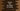# How to find the length of a string in python## How to find the length of a string in python :

We need to find the length of a string most of the time while development of any software. Either you are using python or any other language, finding the string length is one of the most commonly faced problems during software development.

In python, finding the length of a string is really easy. We have one default method to find out the length. We can either calculate the length using a loop or using the library function. In this tutorial, we will learn how to find the length of a string in python using two different ways :

The source code is available here.

## 1. Using a loop :

Using one loop, we can iterate through each character of a string one by one. Initialize one counter variable with initial value as zero. Keep incrementing this counter variable by one for each character while iterating. After the loop will complete, the counter will hold the length of the string.

Note that this method is not recommended to find the length of a string. Because we already have one library method to find it. I will explain it to you later. Let’s try to find the length using a loop first :

### Explanation :

The commented numbers in the above program denote the step numbers below :

1. givenstr_ is the given string. We need to find out the length of this string.
2. Create one integer variable size and assign it a value_ 0_. As mentioned above, this is the counter that will hold the length of the string at the end of the program.
3. Using a for loop, run through each character of the string one by one. For each character, increment the variable size by_ 1_.
4. Finally, print out the size of the string. The variable ‘size’ holds the size of the string. Print its value.

## 2. Using len() :

The main drawback of the above process is that you need to write down the full function anywhere you want to find out the length of a string. For a complete application, we can create one utility class to hold this function. We can call this method from any other class of the application without rewriting it everywhere.

But,instead of writing a for loop to find the length of a string, we can also use one inbuilt method len() to find out the length of a string in python. Let me show you with an example using the same string as shown in the Step-1 :

As you can see that instead of writing a for loop, we can find out the length of a string using the method len(). This is easy to use and more preferable.

### Conclusion :

In this tutorial, we have learned two different ways to find out the length of a string in python. You can go for any of the methods explained above, but the second one i.e. len() is recommended. Try to find the length of different strings and drop one comment below if you have any queries.